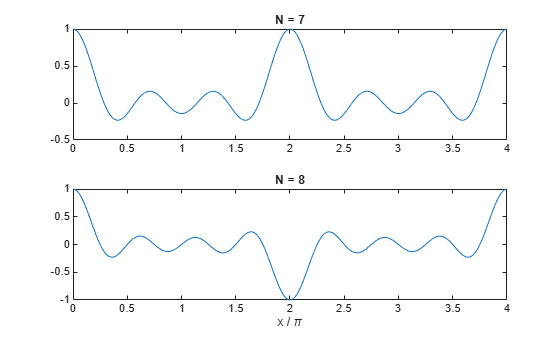# The Dirichlet Function

The function diric computes the Dirichlet function, sometimes called the periodic sinc or aliased sinc function, for an input vector or matrix x. The Dirichlet function is defined by

$D\left(x\right)=\left\{\begin{array}{cc}\frac{\mathrm{sin}\left(Nx/2\right)}{N\mathrm{sin}\left(x/2\right)},& x\ne 2\pi k\text{,}\\ \left(-1{\right)}^{k\left(N-1\right)},& x=2\pi k\text{,}\end{array}\phantom{\rule{1em}{0ex}}k=0,±1,±2,±3,\dots$

where $N$ is a user-specified positive integer. For $N$ odd, the Dirichlet function has a period of $2\pi$; for $N$ even, its period is $4\pi$. The magnitude of this function is $1/N$ times the magnitude of the discrete-time Fourier transform of the $N$-point rectangular window.

To plot the Dirichlet function between 0 and $4\pi$ for $N=7$ and $N=8$, use

x = linspace(0,4*pi,300); subplot(2,1,1) plot(x/pi,diric(x,7)) title('N = 7') subplot(2,1,2) plot(x/pi,diric(x,8)) title('N = 8') xlabel('x / \pi')